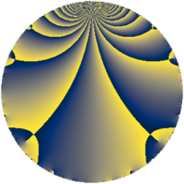# Properties

 Label 1400.1.m.aLevel $1400$ Weight $1$ Character orbit 1400.m Self dual yes Analytic conductor $0.699$ Analytic rank $0$ Dimension $1$ Projective image $D_{2}$ CM/RM discs -7, -56, 8 Inner twists $4$

# Related objects

## Newspace parameters

 Level: $$N$$ $$=$$ $$1400 = 2^{3} \cdot 5^{2} \cdot 7$$ Weight: $$k$$ $$=$$ $$1$$ Character orbit: $$[\chi]$$ $$=$$ 1400.m (of order $$2$$, degree $$1$$, minimal)

## Newform invariants

 Self dual: yes Analytic conductor: $$0.698691017686$$ Analytic rank: $$0$$ Dimension: $$1$$ Coefficient field: $$\mathbb{Q}$$ Coefficient ring: $$\mathbb{Z}$$ Coefficient ring index: $$1$$ Twist minimal: no (minimal twist has level 56) Projective image $$D_{2}$$ Projective field Galois closure of $$\Q(\sqrt{2}, \sqrt{-7})$$ Artin image $D_4$ Artin field Galois closure of 4.0.9800.2

## $q$-expansion

 $$f(q)$$ $$=$$ $$q + q^{2} + q^{4} + q^{7} + q^{8} - q^{9} + O(q^{10})$$ $$q + q^{2} + q^{4} + q^{7} + q^{8} - q^{9} + q^{14} + q^{16} - q^{18} - 2q^{23} + q^{28} + q^{32} - q^{36} - 2q^{46} + q^{49} + q^{56} - q^{63} + q^{64} - 2q^{71} - q^{72} - 2q^{79} + q^{81} - 2q^{92} + q^{98} + O(q^{100})$$

## Character values

We give the values of $$\chi$$ on generators for $$\left(\mathbb{Z}/1400\mathbb{Z}\right)^\times$$.

 $$n$$ $$351$$ $$701$$ $$801$$ $$1177$$ $$\chi(n)$$ $$1$$ $$-1$$ $$-1$$ $$1$$

## Embeddings

For each embedding $$\iota_m$$ of the coefficient field, the values $$\iota_m(a_n)$$ are shown below.

For more information on an embedded modular form you can click on its label.

Label $$\iota_m(\nu)$$ $$a_{2}$$ $$a_{3}$$ $$a_{4}$$ $$a_{5}$$ $$a_{6}$$ $$a_{7}$$ $$a_{8}$$ $$a_{9}$$ $$a_{10}$$
1301.1
 0
1.00000 0 1.00000 0 0 1.00000 1.00000 −1.00000 0
 $$n$$: e.g. 2-40 or 990-1000 Significant digits: Format: Complex embeddings Normalized embeddings Satake parameters Satake angles

## Inner twists

Char Parity Ord Mult Type
1.a even 1 1 trivial
7.b odd 2 1 CM by $$\Q(\sqrt{-7})$$
8.b even 2 1 RM by $$\Q(\sqrt{2})$$
56.h odd 2 1 CM by $$\Q(\sqrt{-14})$$

## Twists

By twisting character orbit
Char Parity Ord Mult Type Twist Min Dim
1.a even 1 1 trivial 1400.1.m.a 1
5.b even 2 1 56.1.h.a 1
5.c odd 4 2 1400.1.c.a 2
7.b odd 2 1 CM 1400.1.m.a 1
8.b even 2 1 RM 1400.1.m.a 1
15.d odd 2 1 504.1.l.a 1
20.d odd 2 1 224.1.h.a 1
35.c odd 2 1 56.1.h.a 1
35.f even 4 2 1400.1.c.a 2
35.i odd 6 2 392.1.j.a 2
35.j even 6 2 392.1.j.a 2
40.e odd 2 1 224.1.h.a 1
40.f even 2 1 56.1.h.a 1
40.i odd 4 2 1400.1.c.a 2
56.h odd 2 1 CM 1400.1.m.a 1
60.h even 2 1 2016.1.l.a 1
80.k odd 4 2 1792.1.c.a 1
80.q even 4 2 1792.1.c.b 1
105.g even 2 1 504.1.l.a 1
105.o odd 6 2 3528.1.bw.a 2
105.p even 6 2 3528.1.bw.a 2
120.i odd 2 1 504.1.l.a 1
120.m even 2 1 2016.1.l.a 1
140.c even 2 1 224.1.h.a 1
140.p odd 6 2 1568.1.n.a 2
140.s even 6 2 1568.1.n.a 2
280.c odd 2 1 56.1.h.a 1
280.n even 2 1 224.1.h.a 1
280.s even 4 2 1400.1.c.a 2
280.ba even 6 2 1568.1.n.a 2
280.bf even 6 2 392.1.j.a 2
280.bi odd 6 2 1568.1.n.a 2
280.bk odd 6 2 392.1.j.a 2
420.o odd 2 1 2016.1.l.a 1
560.be even 4 2 1792.1.c.a 1
560.bf odd 4 2 1792.1.c.b 1
840.b odd 2 1 2016.1.l.a 1
840.u even 2 1 504.1.l.a 1
840.cb even 6 2 3528.1.bw.a 2
840.cg odd 6 2 3528.1.bw.a 2

By twisted newform orbit
Twist Min Dim Char Parity Ord Mult Type
56.1.h.a 1 5.b even 2 1
56.1.h.a 1 35.c odd 2 1
56.1.h.a 1 40.f even 2 1
56.1.h.a 1 280.c odd 2 1
224.1.h.a 1 20.d odd 2 1
224.1.h.a 1 40.e odd 2 1
224.1.h.a 1 140.c even 2 1
224.1.h.a 1 280.n even 2 1
392.1.j.a 2 35.i odd 6 2
392.1.j.a 2 35.j even 6 2
392.1.j.a 2 280.bf even 6 2
392.1.j.a 2 280.bk odd 6 2
504.1.l.a 1 15.d odd 2 1
504.1.l.a 1 105.g even 2 1
504.1.l.a 1 120.i odd 2 1
504.1.l.a 1 840.u even 2 1
1400.1.c.a 2 5.c odd 4 2
1400.1.c.a 2 35.f even 4 2
1400.1.c.a 2 40.i odd 4 2
1400.1.c.a 2 280.s even 4 2
1400.1.m.a 1 1.a even 1 1 trivial
1400.1.m.a 1 7.b odd 2 1 CM
1400.1.m.a 1 8.b even 2 1 RM
1400.1.m.a 1 56.h odd 2 1 CM
1568.1.n.a 2 140.p odd 6 2
1568.1.n.a 2 140.s even 6 2
1568.1.n.a 2 280.ba even 6 2
1568.1.n.a 2 280.bi odd 6 2
1792.1.c.a 1 80.k odd 4 2
1792.1.c.a 1 560.be even 4 2
1792.1.c.b 1 80.q even 4 2
1792.1.c.b 1 560.bf odd 4 2
2016.1.l.a 1 60.h even 2 1
2016.1.l.a 1 120.m even 2 1
2016.1.l.a 1 420.o odd 2 1
2016.1.l.a 1 840.b odd 2 1
3528.1.bw.a 2 105.o odd 6 2
3528.1.bw.a 2 105.p even 6 2
3528.1.bw.a 2 840.cb even 6 2
3528.1.bw.a 2 840.cg odd 6 2

## Hecke kernels

This newform subspace can be constructed as the intersection of the kernels of the following linear operators acting on $$S_{1}^{\mathrm{new}}(1400, [\chi])$$:

 $$T_{3}$$ $$T_{11}$$ $$T_{23} + 2$$ $$T_{113} - 2$$

## Hecke characteristic polynomials

$p$ $F_p(T)$
$2$ $$-1 + T$$
$3$ $$T$$
$5$ $$T$$
$7$ $$-1 + T$$
$11$ $$T$$
$13$ $$T$$
$17$ $$T$$
$19$ $$T$$
$23$ $$2 + T$$
$29$ $$T$$
$31$ $$T$$
$37$ $$T$$
$41$ $$T$$
$43$ $$T$$
$47$ $$T$$
$53$ $$T$$
$59$ $$T$$
$61$ $$T$$
$67$ $$T$$
$71$ $$2 + T$$
$73$ $$T$$
$79$ $$2 + T$$
$83$ $$T$$
$89$ $$T$$
$97$ $$T$$